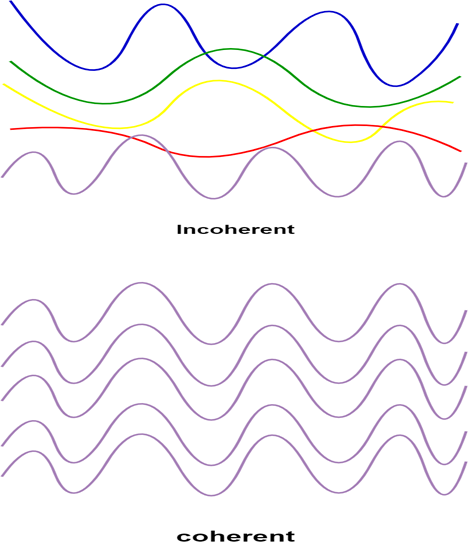Goseeko blog# What are the properties of Laser?

## Introduction

LASER stands for “Light Amplification by Stimulated Emission of Radiation”. Properties of Laser are explained below.

## Properties of Laser

The laser light exhibits some peculiar properties compared with the conventional light which make it unique. Let us study the factors that make laser light stand out. These are

• Monochromatic
• Coherence
• Directionality
•  Highly Intense or Brightness

#### 1. Monochromatic

Monochromatic light is a light containing a single colour or wavelength. The light emitted from ordinary light sources have different energies, frequencies, wavelengths, or colors. But laser light has a single wavelength or colour.

Laser light covers a very narrow range of frequencies or wavelengths. This can be due to the stimulated characteristics of laser light. The bandwidth of the conventional monochromatic light source is 1000 Å. But the bandwidth of an ordinary light source is 10 Å. For a highly sensitive laser source it is 10-8 Å.

#### 2. Coherence

A predictable correlation of the amplitude and phase at any one point with another point is called coherence.That means if two or more waves of same frequency are in the same phase or have constant phase difference then these waves are said to be coherent in nature.

In the case of conventional light, the property of coherence exhibits between a source and its virtual source whereas in the case of laser the property coherence exists between any two or more light waves.There are two types of coherence. Temporal coherence and  Spatial coherence.Figure :Incoherence and Coherence

#### 3. Directionality

The light ray coming from an ordinary light source travels in all directions, but laser light travels in a single direction. For example, the light emitted from torchlight spreads 1km distance it spreads 1 km distance. But the laser light spreads a few centimeters distance even it travels lacks kilometer distance.

The directionality of the laser beam is expressed in terms of divergence

Where r1 and r2 are the radii of laser beam spots at distances of D1 and D2 respectively from the laser source.

#### 4. Highly Intense or Brightness

Laser light is highly intense than conventional light. We know that the intensity of a wave is the energy per unit time flowing through a specific area. A one mill watt He-Ne laser is more intense than the sun intensity. This is because of the coherence and directionality of the laser.

Suppose when two photons each of amplitude a are in phase with another, then young’s principle of superposition, the resultant amplitude of two photons is 2a and the intensity is 4a2. Since in laser many numbers of photons are in phase with each other, the amplitude of the resulting wave becomes na and hence the intensity of the laser is proportional to n2a2. So the 1mW He-Ne laser is more intense than the sun.

Interested in learning about similar topics? Here are a few hand-picked blogs for you!